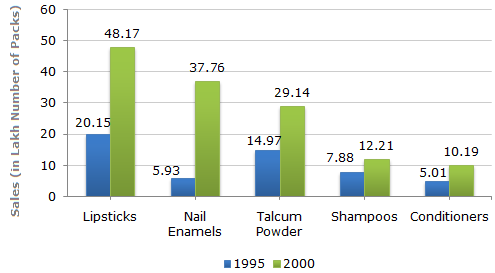# Data Interpretation - Bar Charts

A cosmetic company provides five different products. The sales of these five products (in lakh number of packs) during 1995 and 2000 are shown in the following bar graph.

Sales (in lakh number of packs) of five different products of Cosmetic Company during 1995 and 20001.

The sales of lipsticks in 2000 was by what percent more than the sales of nail enamels in 2000? (rounded off to nearest integer)

 A. 33% B. 31% C. 28% D. 22%

Explanation:

Required percentage
 =(48.17 - 37.76) x 100% 37.76
= 27.57%28%.

2.

During the period 1995-2000, the minimum rate of increase in sales is in the case of?

 A. Shampoos B. Nail enamels C. Talcum powders D. Lipsticks

Explanation:

The percentage increase from 1995 to 2000 for various products are:

 Lipsticks =(48.17 - 20.15) x 100% = 139.06%. 20.15

 Nail enamels =(37.76 - 5.93) x 100% = 536.76%. 5.93

 Talcum powders =(29.14 - 14.97) x 100% = 94.66%. 14.97

 Shampoos =(12.21 - 7.88) x 100% = 54.95%55%. 7.88

 Conditioners =(10.19 - 5.01) x 100% = 103.39%. 5.01The minimum rate of increase in sales from 1995 to 2000 is in the case of Shampoos.

3.

What is the approximate ratio of the sales of nail enamels in 2000 to the sales of Talcum powders in 1995?

 A. 7:2 B. 5:2 C. 4:3 D. 2:1

Explanation:

 Required ratio = 37.762.5 = 5 . 14.97 2

4.

The sales have increase by nearly 55% from 1995 to 2000 in the case of?

 A. Lipsticks B. Nail enamels C. Talcum powders D. Shampoos

Explanation:

The percentage increase from 1995 to 2000 for various products are:

 Lipsticks =(48.17 - 20.15) x 100% = 139.06%. 20.15

 Nail enamels =(37.76 - 5.93) x 100% = 536.76%. 5.93

 Talcum powders =(29.14 - 14.97) x 100% = 94.66%. 14.97

 Shampoos =(12.21 - 7.88) x 100% = 54.95%55%. 7.88

 Conditioners =(10.19 - 5.01) x 100% = 103.39%. 5.01

5.

The sales of conditioners in 1995 was by what percent less than the sales of shampoos in 1995? (rounded off to nearest integer)

 A. 57% B. 36% C. 29% D. 25%

Explanation:

Required percentage
 =(7.88 - 5.01) x 100% 7.88
= 36.42%36%.